Updated on 26 Aug 2021

# What Is Cross-Entropy Loss?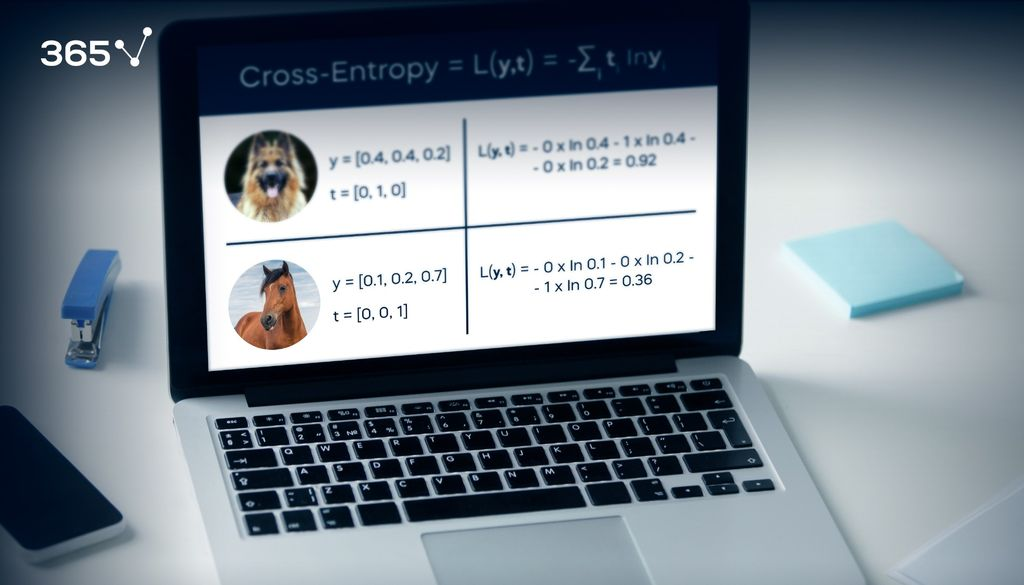Machine learning and deep learning are becoming an increasingly important part of our lives. Whether it’s for business strategy or technological advancement, these techniques help us improve our decision making and future planning. But for that to happen, our models first have to have a high degree of accuracy. That is why loss functions are perhaps the most important part of training your model as they show the accuracy of its performance.

It’s not, however, a one-size-fits-all situation. There are different types of loss function and not all of them will be compatible with your model. Picking the right one is key as this way you ensure that you’re training your model correctly.

In this article, we’ll look at one of the types – namely, the cross-entropy loss function. We’ll explain what it is and provide you with a practical example so you can gain a better understanding of the fundamentals.

## What is Cross-Entropy Loss Function?

Cross-entropy loss refers to the contrast between two random variables; it measures them in order to extract the difference in the information they contain, showcasing the results. We use this type of loss function to calculate how accurate our machine learning or deep learning model is by defining the difference between the estimated probability with our desired outcome.

Essentially, this type of loss function measures your model’s performance by transforming its variables into real numbers, thus, evaluating the “loss” that’s associated with them. The higher the difference between the two, the higher the loss.

We use cross-entropy loss in classification tasks – in fact, it’s the most popular loss function in such cases. And, while the outputs in regression tasks, for example, are numbers, the outputs for classification are categories, like cats and dogs, for example.

Cross-entropy loss is defined as:

$\text{Cross-Entropy} = L\left ( \mathbf{y}, \mathbf{t} \right ) = -\sum_{i} \: \mathbf{t}_{i}\; \ln\mathbf{y}_{i}$

## Cross-Entropy Loss: А Practical Example

Let’s consider these three categories:

• Cats
• Dogs
• Horses

They are all labeled images, with the label acting as the target. But how does it look in numerical terms? Well, the target vector t for this photo would be 0,1,0: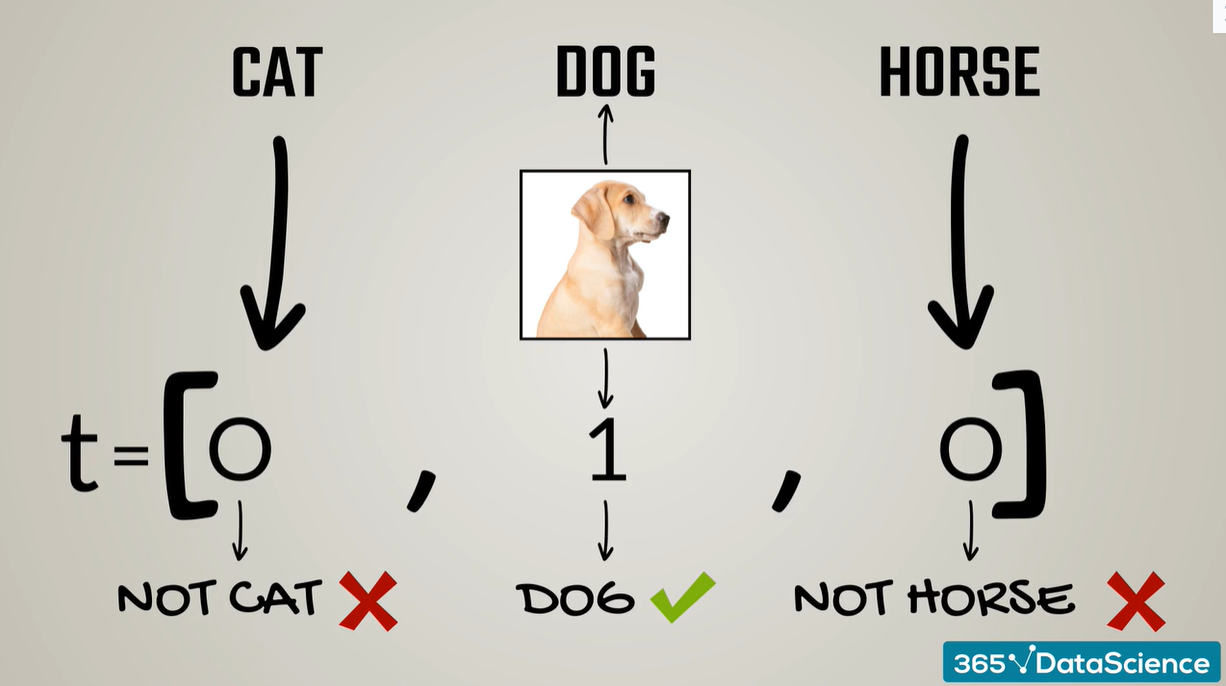The 0s mean it is not a cat or a horse, while the 0 shows it is, indeed, a dog. If we were to examine a picture of a horse, the target vector will be 0,0,1: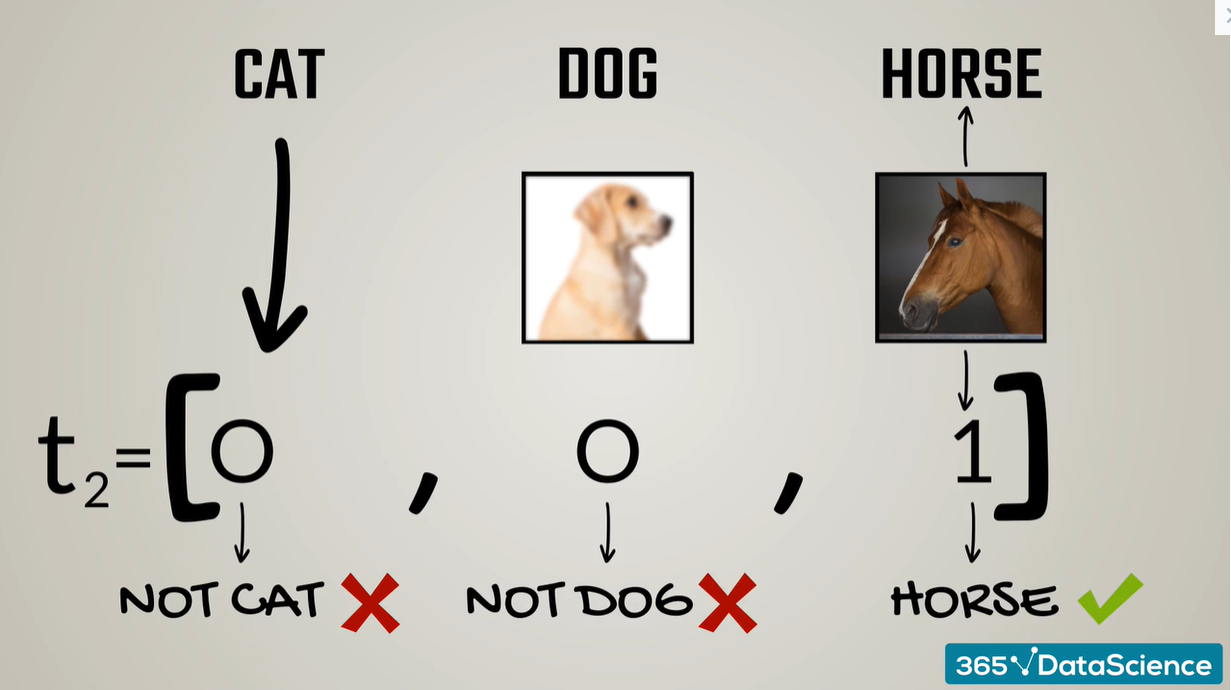Imagine the outputs of our model for these two images are 0.4, 0.4, 0.2 for the first image and 0.1, 0.2, 0.7 for the second: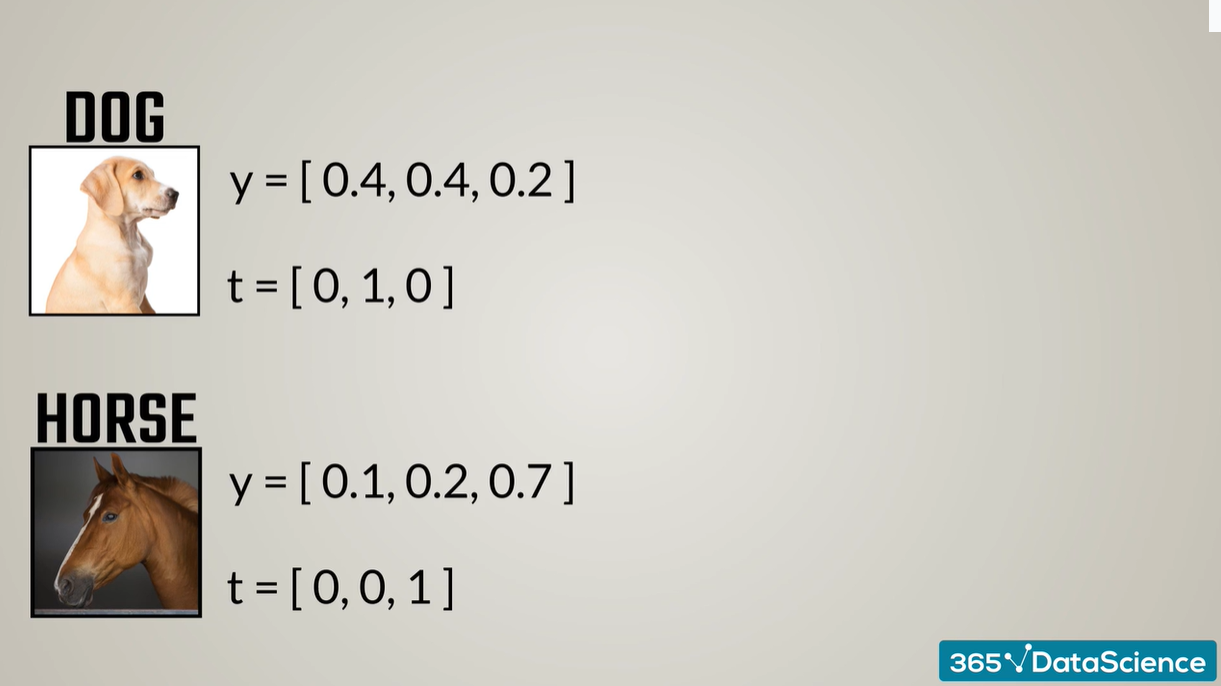After some machine learning transformations, these vectors show the probabilities for each photo being a cat, a dog, or a horse. The first vector shows that, according to our algorithm, there is a 0.4 – or 40% – chance that the first photo is a cat. Following up on that, there is a 40% chance the photo is of a dog and 20% it is of a horse.

What about the cross-entropy of each photo? The loss for the first image would be:

$L(y,t) = -0 \times \ln 0.4 -1 \times \ln 0.4 -0 \times \ln 0.2 = 0.92$

Meanwhile, the cross-entropy loss for the second image is:

$L(y,t) = -0 \times \ln 0.1 -0 \times \ln 0.2 -1 \times \ln 0.7 = 0.36$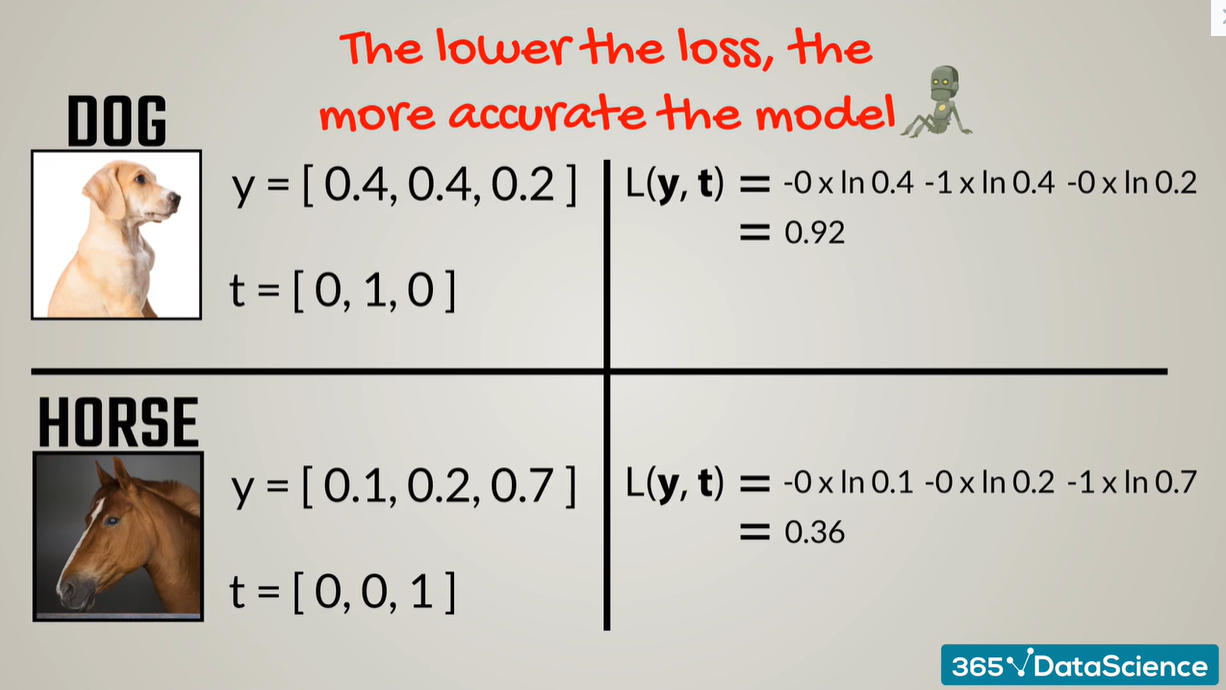As we already know, the lower the loss function, the more accurate the model.

So, what’s the meaning of these two cross-entropies? They show the second loss is lower, therefore, its prediction is superior. For the first image, the model was not sure if the photo was of a dog or a cat – there was an equal 40% probability for both options. We can oppose this to the second photo where the model was 70% sure it was a horse, thus, the cross-entropy was lower.

An important note is that, with classification, our target vectors consist of a bunch of 0s and a 1 which indicates the correct category. Therefore, we could simplify the above formulas to: minus the log of the probability of the output for the correct answer.

Here’s an illustration of how our initial formulas would change: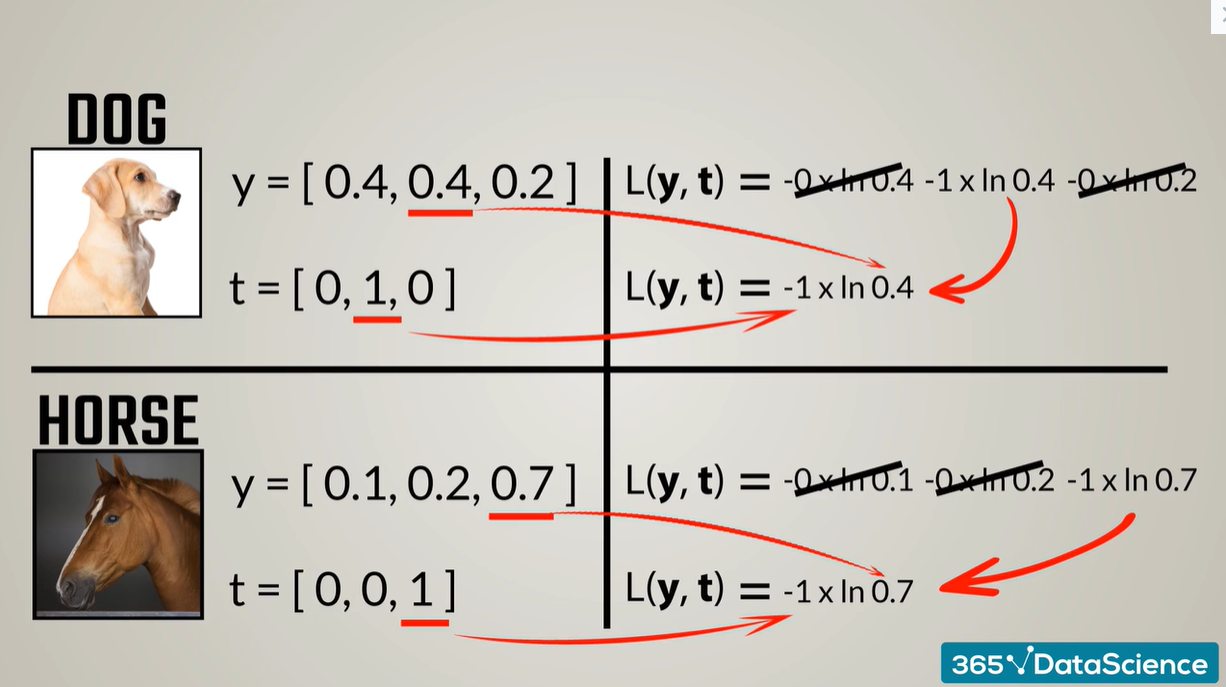## Cross-Entropy Loss Function: Next Steps

It’s no surprise that cross-entropy loss is the most popular function used in machine learning or deep learning classification. After all, it helps determine the accuracy of our model in numerical values – 0s and 1s, which we can later extract the probability percentage from.

There are, of course, other loss functions that can help us resolve a problem. We must emphasize that any function that holds the basic property of being higher for worse results and lower for better results can be a loss function.

These loss functions are quite important as they help us improve the accuracy of our models significantly. So, if you want to gain a better understanding of cross-entropy loss, as well as other types of loss function, we can help you start your machine learning and deep learning journey for free.